# Python Learning Week 8 (Solution)

Write a program to implement KNN classifier and classify given vector. (for k = 3)

CODE: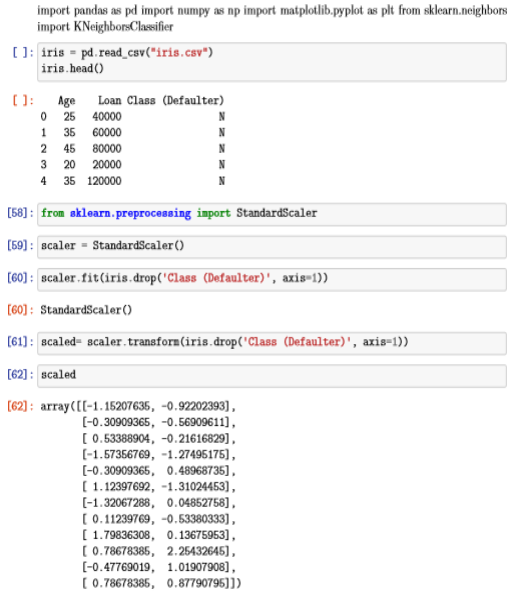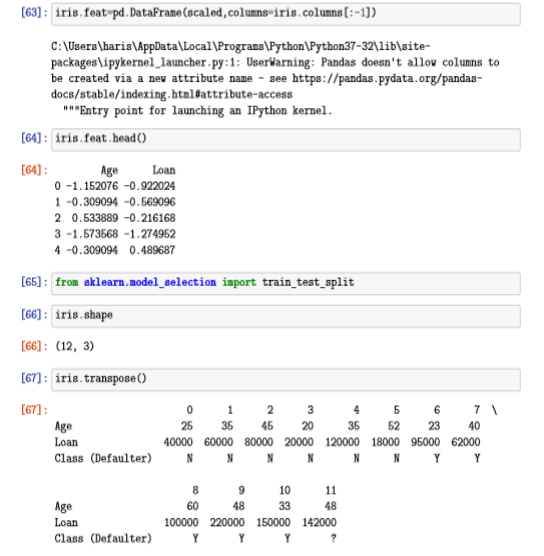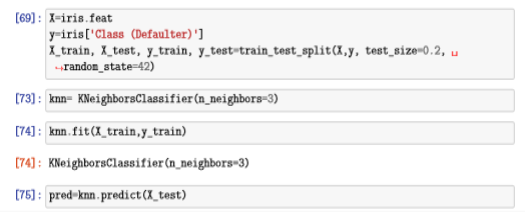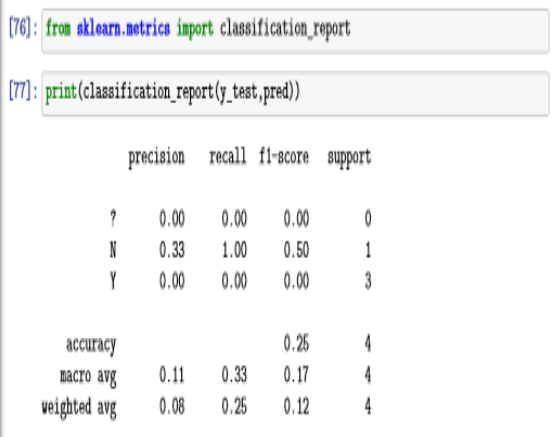Modify Task 1  and perform it for all odd values of k from 1 to 10.

• Find accuracy for each value of k and display.
• Find the best k which gives highest value of accuracy
• Also compute confusion matrix for the best value of k.

CODE: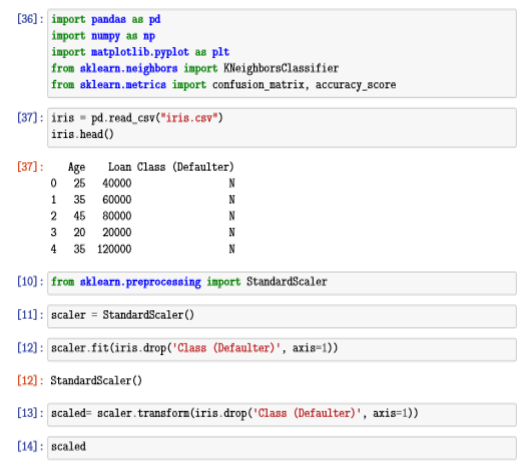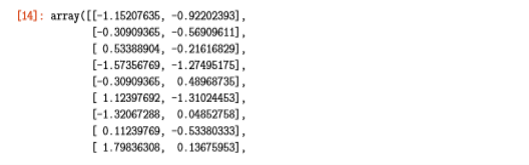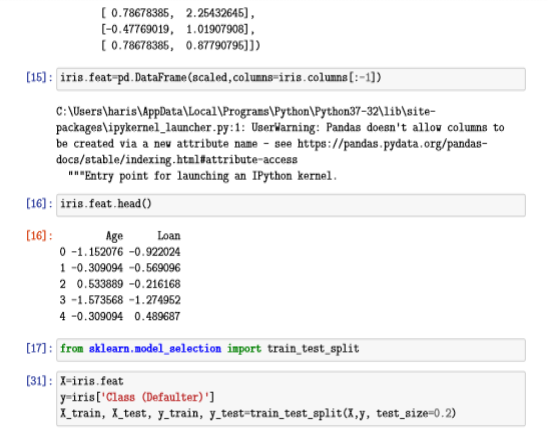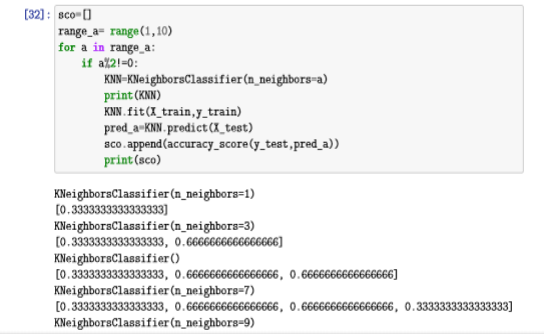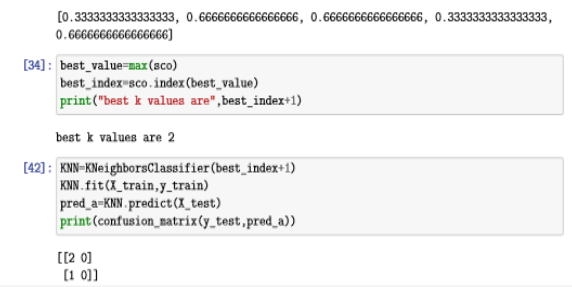What we studied in Week 8:
In this week we study K-Nearest Neighbor Classification Model using built in function. Basically K-Nearest Neighbors is one of the most basic machine learning algorithm. This machine learning algorithm is one of the types of machine learning algorithm which is dependent on labelled input data in order to learn a function which is capable of producing an output whenever a new unlabeled data is given as input. K-NN is widely used in real life scenarios, as it is non-parametric which means that it does not make any underlying assumptions about the distributions of data and also implement this algorithm in python. This is all we have studied in this week.